Approximate time: 80 minutes

## Learning Objectives

• Transforming counts for unsupervised clustering methods
• Evaluating quality of samples using Principal Components Analysis
• Hierarchical clustering of samples in the dataset

# Quality Control

The next step in the DESeq2 workflow is QC, which includes sample-level and gene-level steps to perform QC checks on the count data to help us ensure that the samples/replicates look good.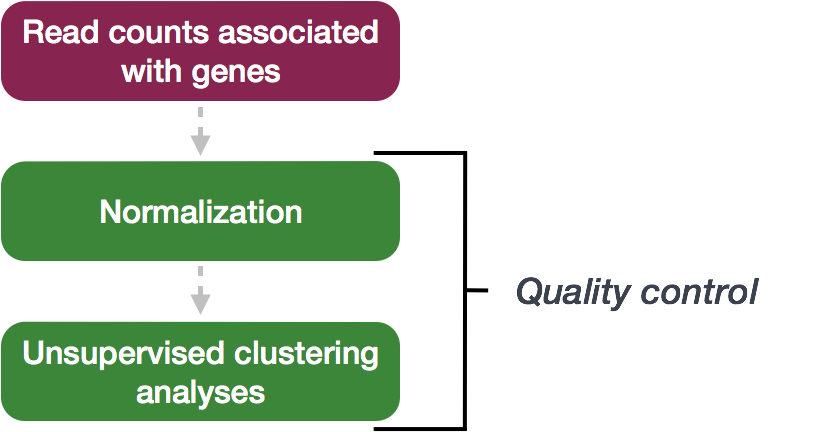## Sample-level QC

A useful initial step in an RNA-seq analysis is often to assess overall similarity between samples:

• Which samples are similar to each other, which are different?
• Does this fit to the expectation from the experiment’s design?
• What are the major sources of variation in the dataset?

To explore the similarity of our samples, we will be performing sample-level QC using Principal Component Analysis (PCA) and hierarchical clustering methods. Our sample-level QC allows us to see how well our replicates cluster together, as well as, observe whether our experimental condition represents the major source of variation in the data. Performing sample-level QC can also identify any sample outliers, which may need to be explored further to determine whether they need to be removed prior to DE analysis.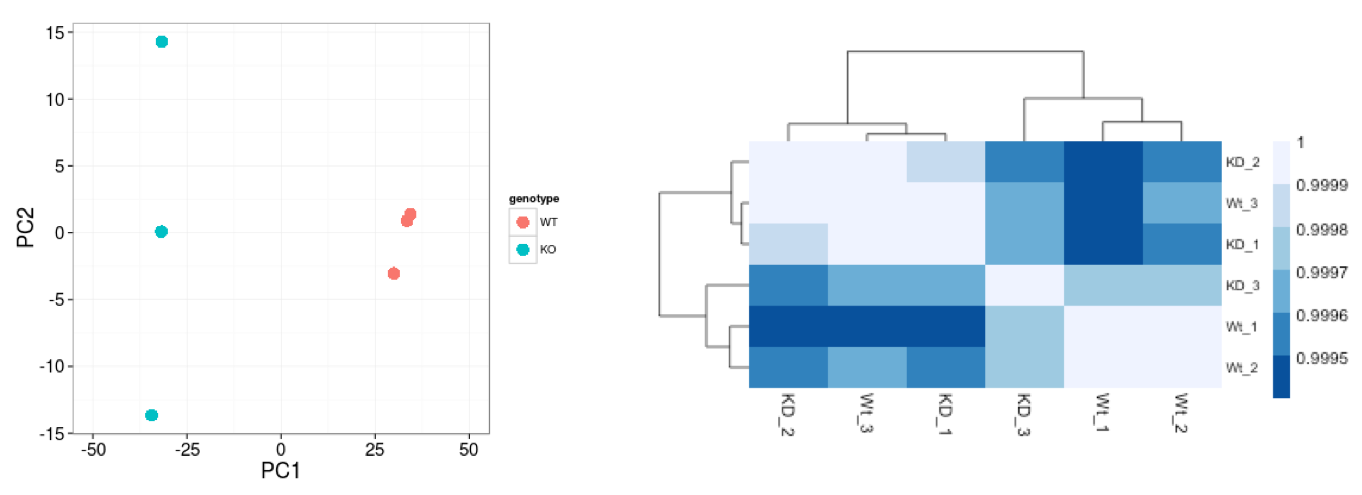When using these unsupervised clustering methods, log2-transformation of the normalized counts improves the distances/clustering for visualization. DESeq2 uses a regularized log transform (rlog) of the normalized counts for sample-level QC as it moderates the variance across the mean, improving the clustering.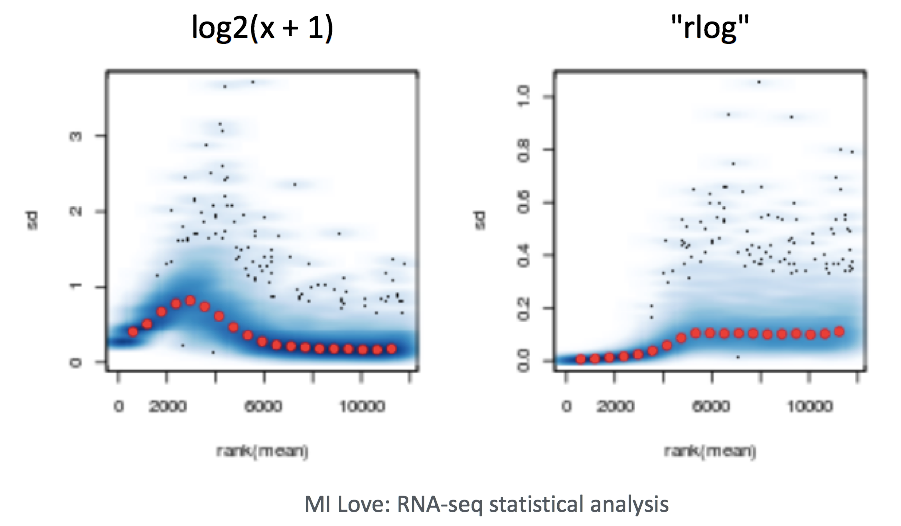### Principal Component Analysis (PCA)

Principal Component Analysis (PCA) is a technique used to emphasize variation and bring out strong patterns in a dataset (dimensionality reduction). Details regarding PCA are given below (based on materials from StatQuest, and if you would like a more thorough description, we encourage you to explore StatQuest’s video.

Suppose we had a dataset with two samples and four genes. Based on this expression data we want to evaluate the relationship between these samples. We could plot the counts of one sample versus another, with Sample 1 on the x-axis and Sample 2 on the y-axis as shown below: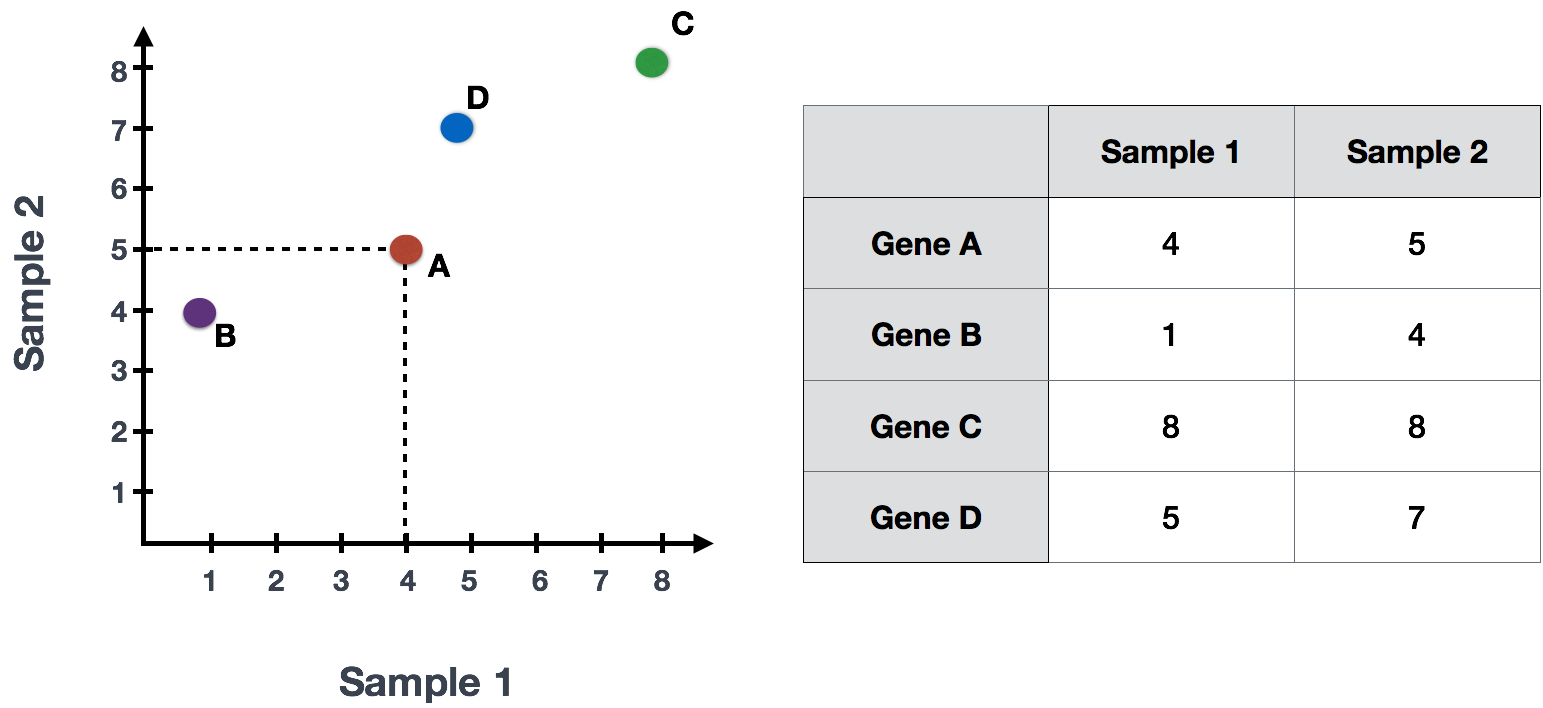For PCA analysis, the first step is taking this plot and drawing a line through the data in the direction representing the most variation. In this example, the most variation is along the diagonal. That is, the largest spread in the data is between the two endpoints of this line. This is called the first principal component, or PC1. The genes at the endpoints of this line (Gene B and Gene C) have the greatest influence on the direction of this line.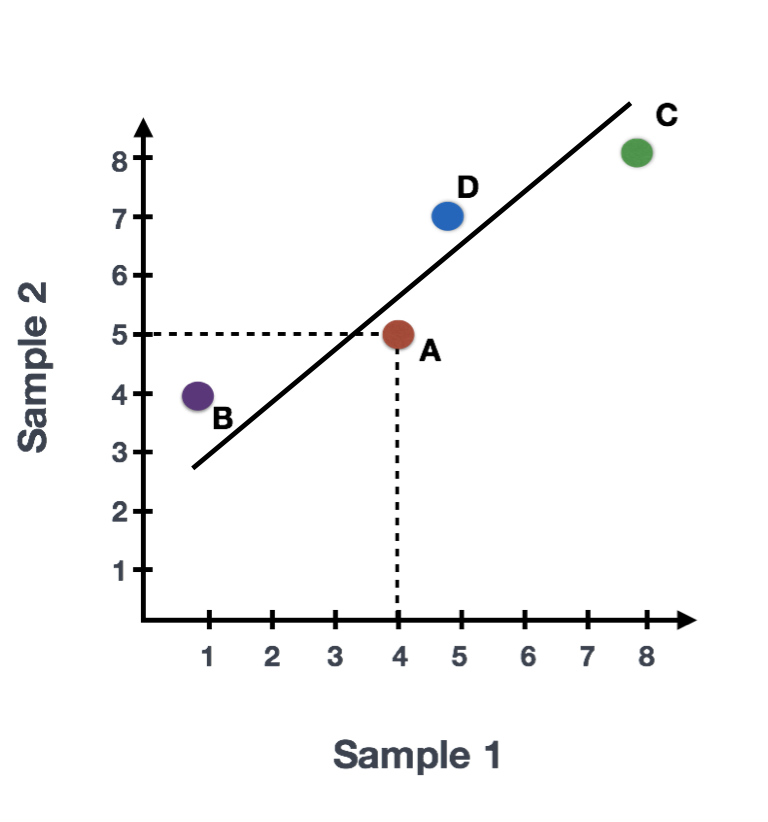After drawing this line and establishing the amount of influence per gene, PCA will compute a per sample score. The per sample PC1 score is computed by taking the product of the influence and the normalized read count and summing across all genes. We could draw another line through the data representing the second most amount of variation in the data (PC2) and compute scores, followed by a third line and so on until you hit the total number of samples in your dataset.

``````Sample1 PC1 score = (read count Gene A * influence Gene A) + (read count Gene B * influence Gene B) + .. for all genes
``````

In reality, your dataset will have larger dimensions (more samples, and many, many more genes). The initial sample-to-sample plot, will therefore be in n-dimensional space with n axes representing the total number of samples you have. The end result is a 2-dimensional matrix with rows representing samples and columns reflecting scores for each of the principal components. To evaluate the results of a PCA, we usually plot principal components against each other, starting with PCs that explain the most amount of variation in your data.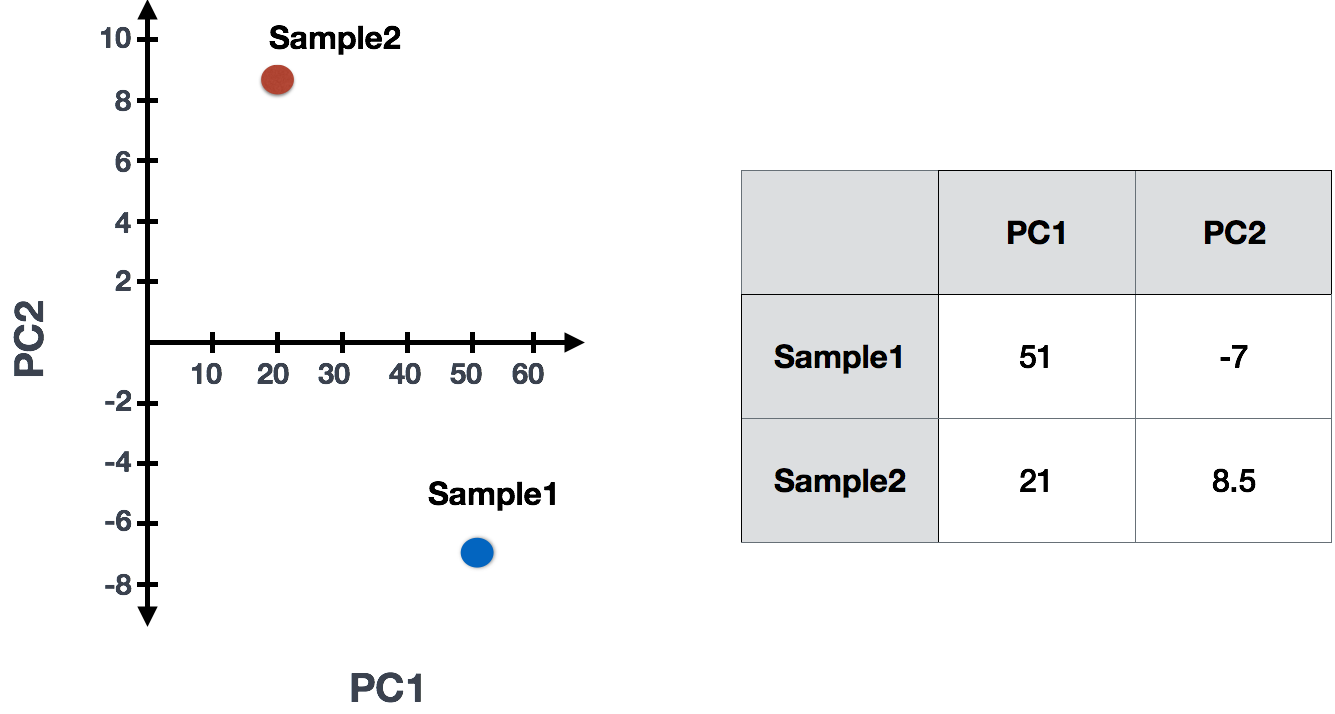If two samples have similar levels of expression for the genes that contribute significantly to the variation represented by PC1, they will be plotted close together on the PC1 axis. Therefore, we would expect that biological replicates to have similar scores (since the same genes are changing) and cluster together on PC1 and/or PC2, and the samples from different treatment groups to have different score. This is easiest to understand by visualizing example PCA plots.

#### Interpreting PCA plots

We have an example dataset and a few associated PCA plots below to get a feel for how to interpret them. The metadata for the experiment is displayed below. The main condition of interest is `treatment`.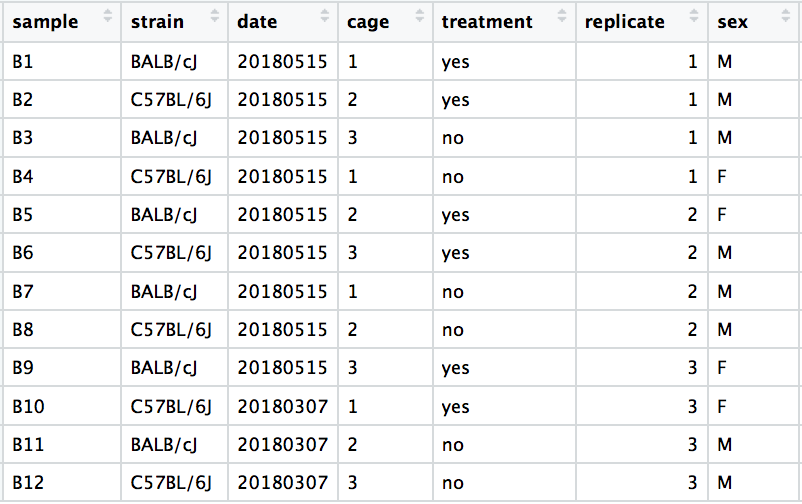When visualizing on PC1 and PC2, we don’t see the samples separate by `treatment`, so we decide to explore other sources of variation present in the data. We hope that we have included all possible known sources of variation in our metadata table, and we can use these factors to color the PCA plot.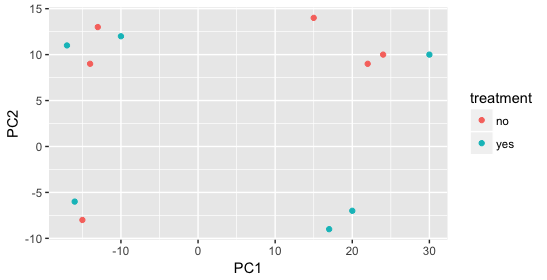We start with the factor `cage`, but the `cage` factor does not seem to explain the variation on PC1 or PC2.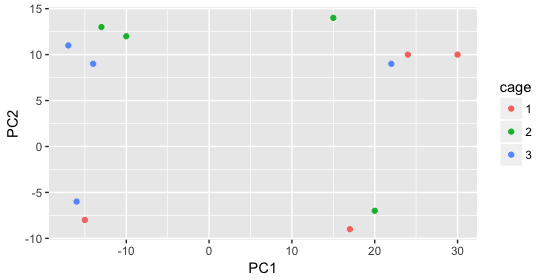Then, we color by the `sex` factor, which appears to separate samples on PC2. This is good information to take note of, as we can use it downstream to account for the variation due to sex in the model and regress it out.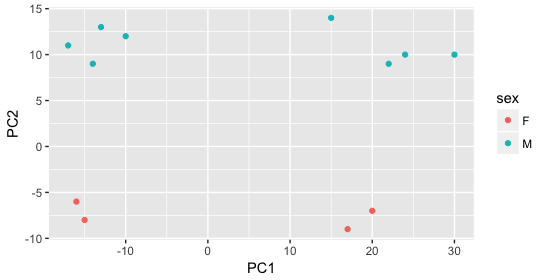Next we explore the `strain` factor and find that it explains the variation on PC1.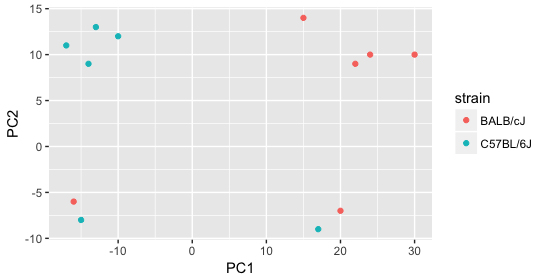It’s great that we have been able to identify the sources of variation for both PC1 and PC2. By accounting for it in our model, we should be able to detect more genes differentially expressed due to `treatment`.

Worrisome about this plot is that we see two samples that do not cluster with the correct strain. This would indicate a likely sample swap and should be investigated to determine whether these samples are indeed the labeled strains. If we found there was a switch, we could swap the samples in the metadata. However, if we think they are labeled correctly or are unsure, we could just remove the samples from the dataset.

Still we haven’t found if `treatment` is a major source of variation after `strain` and `sex`. So, we explore PC3 and PC4 to see if `treatment` is driving the variation represented by either of these PCs.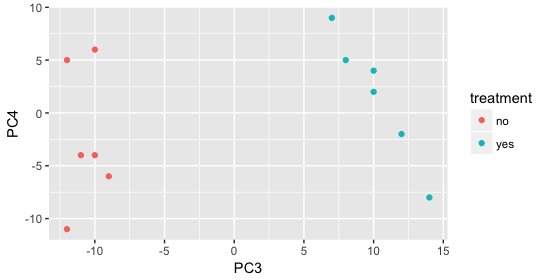We find that the samples separate by `treatment` on PC3, and are optimistic about our DE analysis since our condition of interest, `treatment`, is separating on PC3 and we can regress out the variation driving PC1 and PC2.

Depending on how much variation is explained by the first few principal components, you may want to explore more (i.e consider more components and plot pairwise combinations). Even if your samples do not separate clearly by the experimental variable, you may still get biologically relevant results from the DE analysis. If you are expecting very small effect sizes, then it’s possible the signal is drowned out by extraneous sources of variation. In situations where you can identify those sources, it is important to account for these in your model, as it provides more power to the tool for detecting DE genes.

Exercise

The figure below was generated from a time course experiment with sample groups ‘Ctrl’ and ‘Sci’ and the following timepoints: 0h, 2h, 8h, and 16h.

• Determine the sources explaining the variation represented by PC1 and PC2.
• Do the sample groups separate well?
• Do the replicates cluster together for each sample group?
• Are there any outliers in the data?
• Should we have any other concerns regarding the samples in the dataset?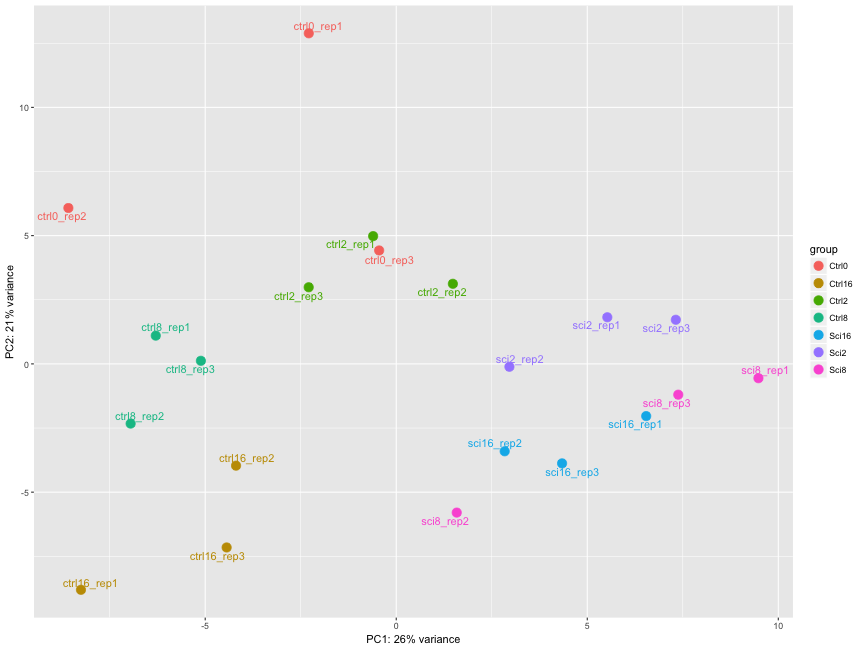### Hierarchical Clustering Heatmap

Similar to PCA, hierarchical clustering is another, complementary method for identifying strong patterns in a dataset and potential outliers. The heatmap displays the correlation of gene expression for all pairwise combinations of samples in the dataset. Since the majority of genes are not differentially expressed, samples generally have high correlations with each other (values higher than 0.80). Samples below 0.80 may indicate an outlier in your data and/or sample contamination.

The hierarchical tree can indicate which samples are more similar to each other based on the normalized gene expression values. The color blocks indicate substructure in the data, and you would expect to see your replicates cluster together as a block for each sample group. Additionally, we expect to see samples clustered similar to the groupings observed in a PCA plot.

In the plot below, we would be quite concerned about ‘Wt_3’ and ‘KO_3’ samples not clustering with the other replicates. We would want to explore the PCA to see if we see the same clustering of samples.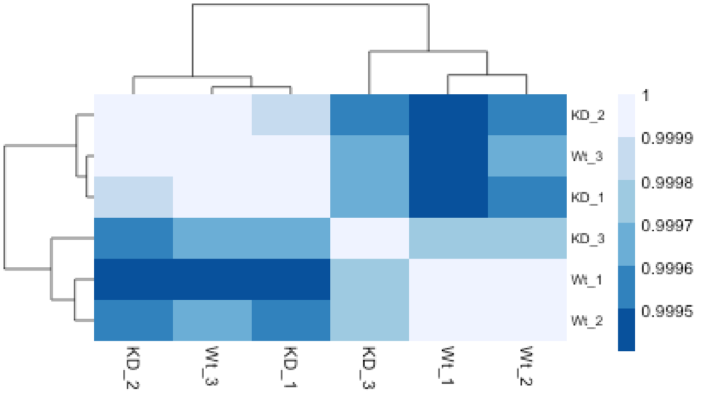## Gene-level QC

In addition to examining how well the samples/replicates cluster together, there are a few more QC steps. Prior to differential expression analysis it is beneficial to omit genes that have little or no chance of being detected as differentially expressed. This will increase the power to detect differentially expressed genes. The genes omitted fall into three categories:

• Genes with zero counts in all samples
• Genes with an extreme count outlier
• Genes with a low mean normalized counts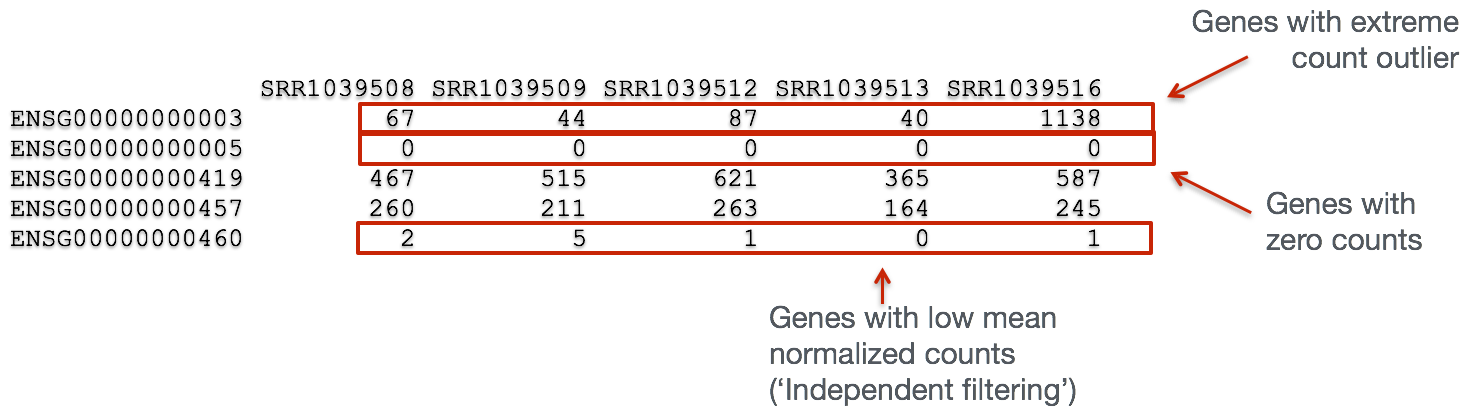DESeq2 will perform this filtering by default; however other DE tools, such as EdgeR will not. Filtering is a necessary step, even if you are using limma-voom and/or edgeR’s quasi-likelihood methods. Be sure to follow pre-filtering steps when using other tools, as outlined in their user guides found on Bioconductor as they generally perform much better.

## Mov10 quality assessment and exploratory analysis using DESeq2

Now that we have a good understanding of the QC steps normally employed for RNA-seq, let’s implement them for the Mov10 dataset we are going to be working with.

### Transform normalized counts using the rlog transformation

To improve the distances/clustering for the PCA and heirarchical clustering visualization methods, we need to moderate the variance across the mean by applying the rlog transformation to the normalized counts.

The rlog transformation of the normalized counts is only necessary for these visualization methods during this quality assessment. We will not be using these tranformed counts downstream.

``````### Transform counts for data visualization
rld <- rlog(dds, blind=TRUE)
``````

The `blind=TRUE` argument results in a transformation unbiased to sample condition information. When performing quality assessment, it is important to include this option. The DESeq2 vignette has more details.

The `rlog` function returns a `DESeqTransform` object, another type of DESeq-specific object. The reason you don’t just get a matrix of transformed values is because all of the parameters (i.e. size factors) that went into computing the rlog transform are stored in that object. We use this object to plot the PCA and heirarchical clustering figures for quality assessment.

NOTE: The `rlog()` funtion can be a bit slow when you have e.g. > 20 samples. In these situations the `vst()` function is much faster and performs a similar transformation appropriate for use with `plotPCA()`. It’s typically just a few seconds with `vst()` due to optimizations and the nature of the transformation.

### Principal components analysis (PCA)

DESeq2 has a built-in function for plotting PCA plots, that uses `ggplot2` under the hood. This is great because it saves us having to type out lines of code and having to fiddle with the different ggplot2 layers. In addition, it takes the `rlog` object as an input directly, hence saving us the trouble of extracting the relevant information from it.

The function `plotPCA()` requires two arguments as input: an `rlog` object and the `intgroup` (the column in our metadata that we are interested in).

``````### Plot PCA
plotPCA(rld, intgroup="sampletype")
``````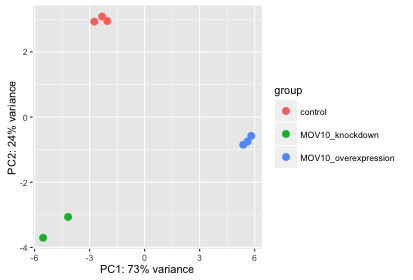What does this plot tell you about the similarity of samples? Does it fit the expectation from the experimental design? By default the function uses the top 500 most variable genes. You can change this by adding the `ntop` argument and specifying how many genes you want to use to draw the plot.

NOTE: The `plotPCA()` function will only return the values for PC1 and PC2. If you would like to explore the additional PCs in your data or if you would like to identify genes that contribute most to the PCs, you can use the `prcomp()` function. For example, to plot any of the PCs we could run the following code:

`````` # Input is a matrix of log transformed values
rld <- rlog(dds, blind=T)
rld_mat <- assay(rld)
pca <- prcomp(t(rld_mat))

# Create data frame with metadata and PC3 and PC4 values for input to ggplot
df <- cbind(meta, pca\$x)
ggplot(df) + geom_point(aes(x=PC3, y=PC4, color = sampletype))
``````

Resources are available to learn how to do more complex inquiries using the PCs.

### Hierarchical Clustering

Since there is no built-in function for heatmaps in DESeq2 we will be using the `pheatmap()` function from the `pheatmap` package. This function requires a matrix/dataframe of numeric values as input, and so the first thing we need to is retrieve that information from the `rld` object:

``````### Extract the rlog matrix from the object
rld_mat <- assay(rld)    ## assay() is function from the "SummarizedExperiment" package that was loaded when you loaded DESeq2
``````

Then we need to compute the pairwise correlation values for samples. We can do this using the `cor()` function:

``````### Compute pairwise correlation values
rld_cor <- cor(rld_mat)    ## cor() is a base R function

head(rld_cor)   ## check the output of cor(), make note of the rownames and colnames
``````

And now to plot the correlation values as a heatmap:

``````### Plot heatmap
pheatmap(rld_cor)
``````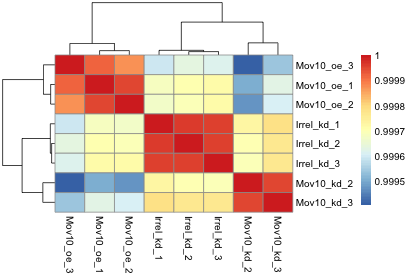Overall, we observe pretty high correlations across the board ( > 0.999) suggesting no outlying sample(s). Also, similar to the PCA plot you see the samples clustering together by sample group. Together, these plots suggest to us that the data are of good quality and we have the green light to proceed to differential expression analysis.

NOTE: The `pheatmap` function has a number of different arguments that we can alter from default values to enhance the aesthetics of the plot. If you are curious and want to explore more, try running the code below. How does your plot change? Take a look through the help pages (`?pheatmap`) and identify what each of the added arguments is contributing to the plot.

``````heat.colors <- brewer.pal(6, "Blues")
pheatmap(rld_cor, color = heat.colors, border_color=NA, fontsize = 10,
fontsize_row = 10, height=20)
``````

Curious on all of the available color palettes offered by the RColorBrewer package? Try typing in your console `display.brewer.all()` and see what happens!

This lesson has been developed by members of the teaching team at the Harvard Chan Bioinformatics Core (HBC). These are open access materials distributed under the terms of the Creative Commons Attribution license (CC BY 4.0), which permits unrestricted use, distribution, and reproduction in any medium, provided the original author and source are credited.